#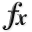N-D Test Functions R¶

class go_benchmark.Rana(dimensions=2)

Rana test objective function.

This class defines the Rana global optimization problem. This is a multimodal minimization problem defined as follows: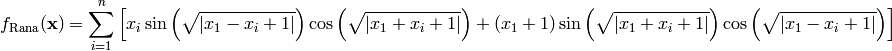Here,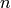represents the number of dimensions and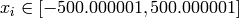for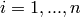.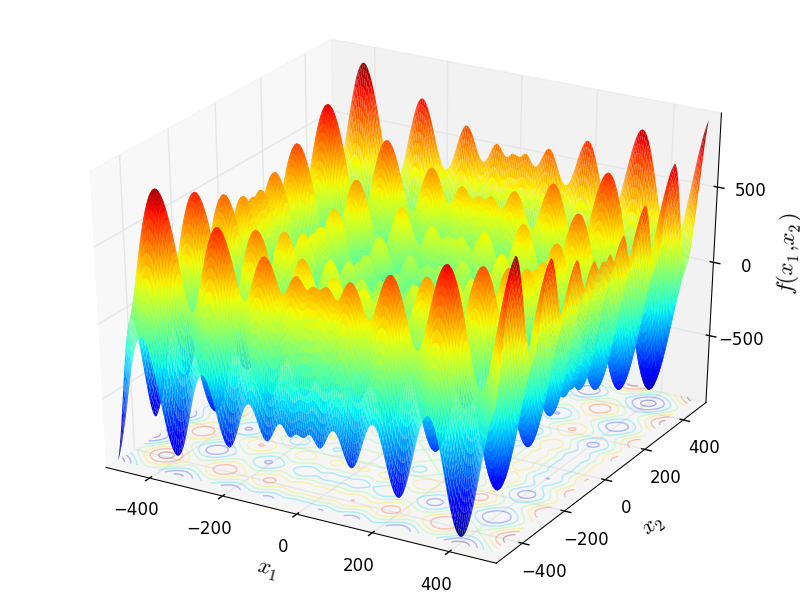Two-dimensional Rana function

Global optimum: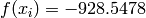forforclass go_benchmark.Rastrigin(dimensions=2)

Rastrigin test objective function.

This class defines the Rastrigin global optimization problem. This is a multimodal minimization problem defined as follows:Here,represents the number of dimensions and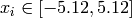for.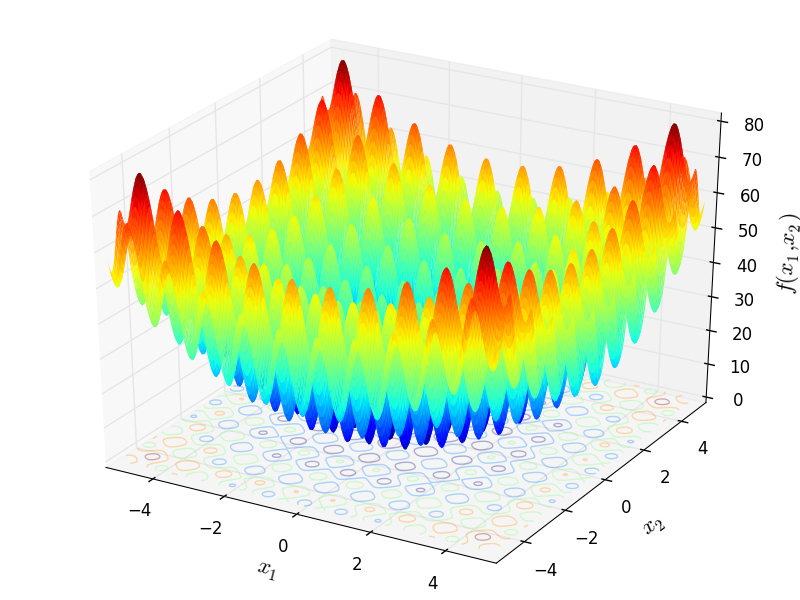Two-dimensional Rastrigin function

Global optimum: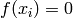for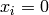forclass go_benchmark.Ripple01(dimensions=2)

Ripple 1 test objective function.

This class defines the Ripple 1 global optimization problem. This is a multimodal minimization problem defined as follows: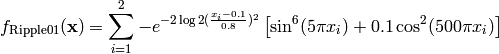Here,represents the number of dimensions and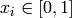for.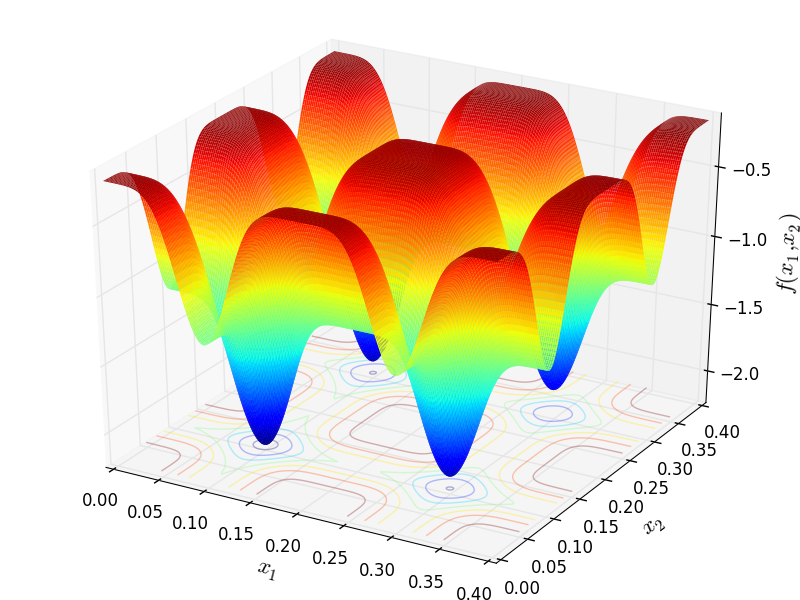Two-dimensional Ripple 1 function

Global optimum: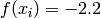for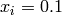for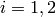class go_benchmark.Ripple25(dimensions=2)

Ripple 25 test objective function.

This class defines the Ripple 25 global optimization problem. This is a multimodal minimization problem defined as follows: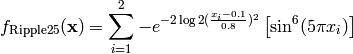Here,represents the number of dimensions andfor.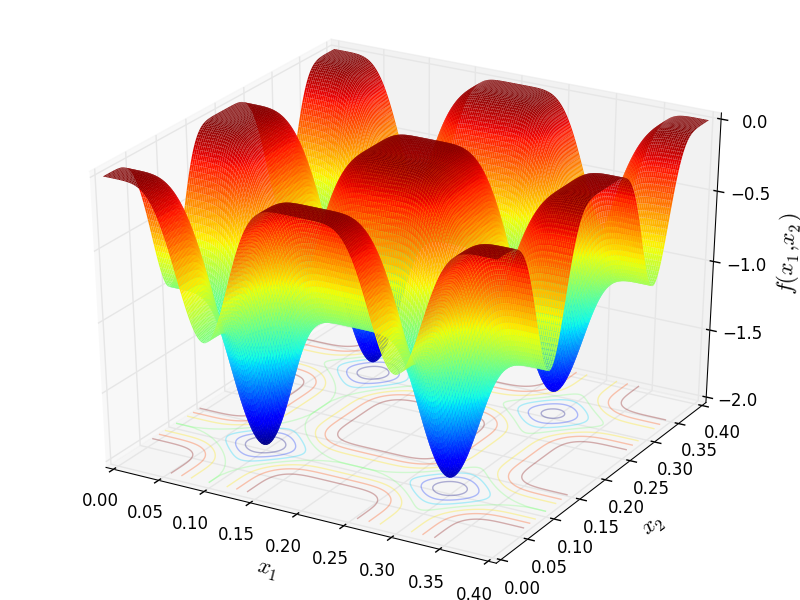Two-dimensional Ripple 25 function

Global optimum: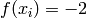forforclass go_benchmark.Rosenbrock(dimensions=2)

Rosenbrock test objective function.

This class defines the Rosenbrock global optimization problem. This is a multimodal minimization problem defined as follows: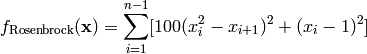Here,represents the number of dimensions andfor.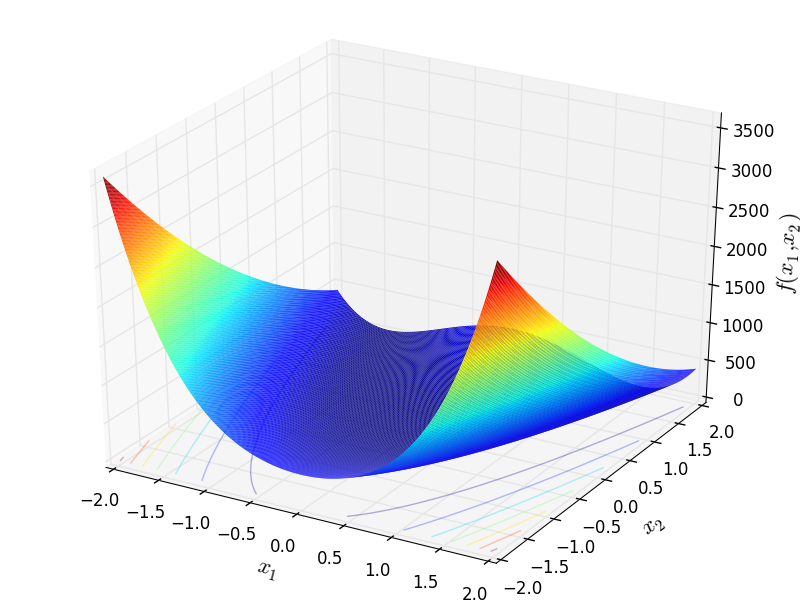Two-dimensional Rosenbrock function

Global optimum:for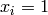forclass go_benchmark.RosenbrockModified(dimensions=2)

Modified Rosenbrock test objective function.

This class defines the Modified Rosenbrock global optimization problem. This is a multimodal minimization problem defined as follows:Here,represents the number of dimensions and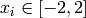for.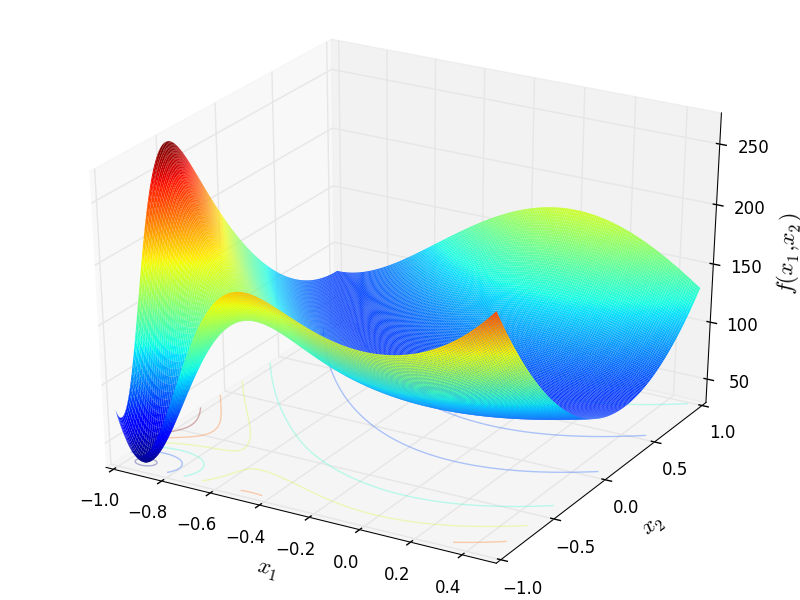Two-dimensional Modified Rosenbrock function

Global optimum:for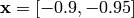class go_benchmark.RotatedEllipse01(dimensions=2)

Rotated Ellipse 1 test objective function.

This class defines the Rotated Ellipse 1 global optimization problem. This is a unimodal minimization problem defined as follows: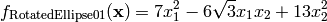Here,represents the number of dimensions and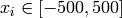for.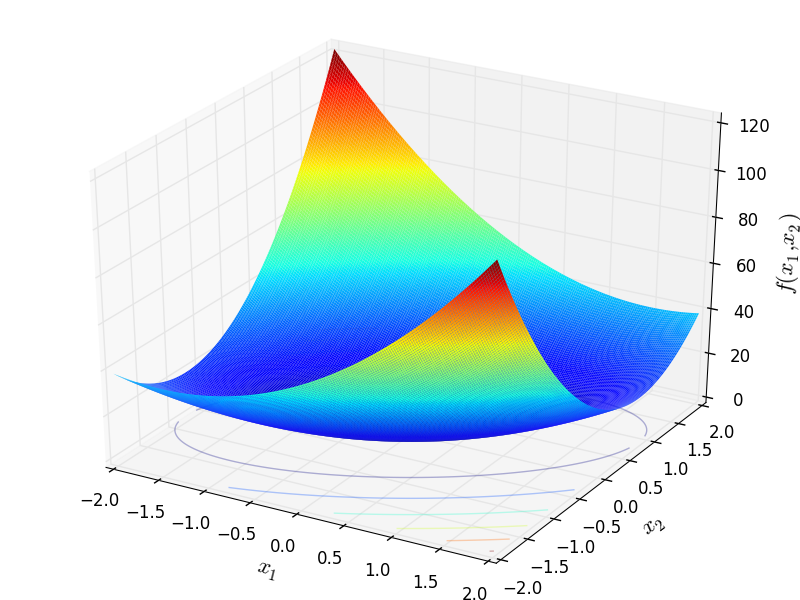Two-dimensional Rotated Ellipse 1 function

Global optimum:forclass go_benchmark.RotatedEllipse02(dimensions=2)

Rotated Ellipse 2 test objective function.

This class defines the Rotated Ellipse 2 global optimization problem. This is a unimodal minimization problem defined as follows: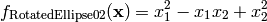Here,represents the number of dimensions andfor.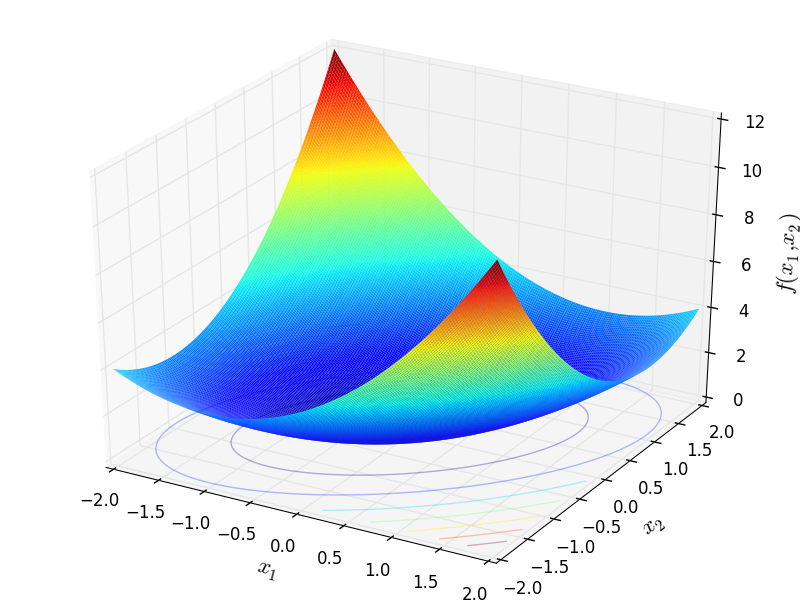Two-dimensional Rotated Ellipse 2 function

Global optimum:for#### Previous topic

N-D Test Functions Q

#### Next topic

N-D Test Functions S